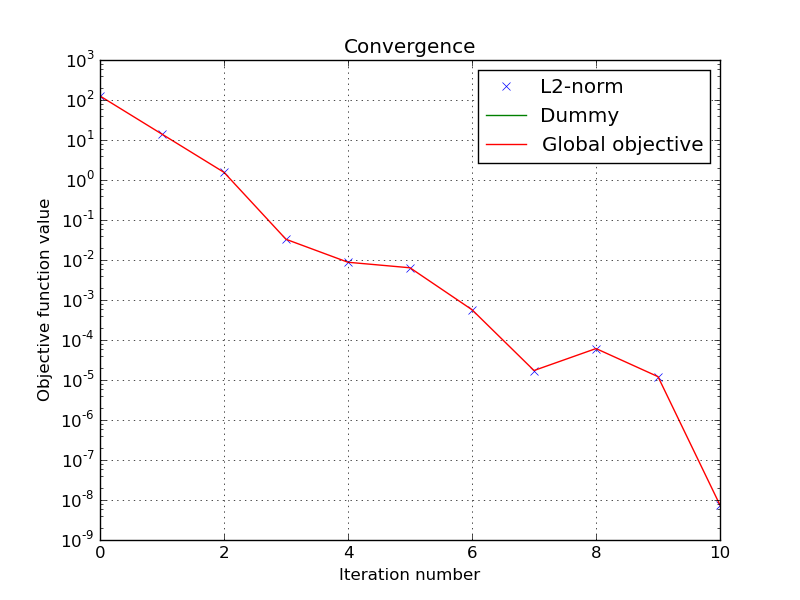# Simple least square problem¶

This simplistic example is only meant to demonstrate the basic workflow of the toolbox. Here we want to solve a least square problem, i.e. we want the solution to converge to the original signal without any constraint. Lets define this signal by :

>>> y = [4, 5, 6, 7]


The first function to minimize is the sum of squared distances between the current signal x and the original y. For this purpose, we instantiate an L2-norm object :

>>> from pyunlocbox import functions
>>> f1 = functions.norm_l2(y=y)


This standard function object provides the eval(), grad() and prox() methods that will be useful to the solver. We can evaluate them at any given point :

>>> f1.eval([0, 0, 0, 0])
126
array([ -8, -10, -12, -14])
>>> f1.prox([0, 0, 0, 0], 1)
array([ 2.66666667,  3.33333333,  4.        ,  4.66666667])


We need a second function to minimize, which usually describes a constraint. As we have no constraint, we just define a dummy function object by hand. We have to define the _eval() and _grad() methods as the solver we will use requires it :

>>> f2 = functions.func()
>>> f2._eval = lambda x: 0
>>> f2._grad = lambda x: 0


Note

We could also have used the pyunlocbox.functions.dummy function object.

We can now instantiate the solver object :

>>> from pyunlocbox import solvers
>>> solver = solvers.forward_backward()


And finally solve the problem :

>>> x0 = [0., 0., 0., 0.]
>>> ret = solvers.solve([f2, f1], x0, solver, atol=1e-5, verbosity='HIGH')
func evaluation : 0.000000e+00
norm_l2 evaluation : 1.260000e+02
INFO: Forward-backward method : FISTA
Iteration 1 of forward_backward :
func evaluation : 0.000000e+00
norm_l2 evaluation : 1.400000e+01
objective = 1.40e+01
Iteration 2 of forward_backward :
func evaluation : 0.000000e+00
norm_l2 evaluation : 1.555556e+00
objective = 1.56e+00
Iteration 3 of forward_backward :
func evaluation : 0.000000e+00
norm_l2 evaluation : 3.293044e-02
objective = 3.29e-02
Iteration 4 of forward_backward :
func evaluation : 0.000000e+00
norm_l2 evaluation : 8.780588e-03
objective = 8.78e-03
Iteration 5 of forward_backward :
func evaluation : 0.000000e+00
norm_l2 evaluation : 6.391406e-03
objective = 6.39e-03
Iteration 6 of forward_backward :
func evaluation : 0.000000e+00
norm_l2 evaluation : 5.713369e-04
objective = 5.71e-04
Iteration 7 of forward_backward :
func evaluation : 0.000000e+00
norm_l2 evaluation : 1.726501e-05
objective = 1.73e-05
Iteration 8 of forward_backward :
func evaluation : 0.000000e+00
norm_l2 evaluation : 6.109470e-05
objective = 6.11e-05
Iteration 9 of forward_backward :
func evaluation : 0.000000e+00
norm_l2 evaluation : 1.212636e-05
objective = 1.21e-05
Iteration 10 of forward_backward :
func evaluation : 0.000000e+00
norm_l2 evaluation : 7.460428e-09
objective = 7.46e-09
Solution found after 10 iterations :
objective function f(sol) = 7.460428e-09
stopping criterion : ATOL


The solving function returns several values, one is the found solution :

>>> ret['sol']
array([ 3.99996922,  4.99996153,  5.99995383,  6.99994614])


Another one is the value returned by each function objects at each iteration. As we passed two function objects (L2-norm and dummy), the objective is a 2 by 11 (10 iterations plus the evaluation at x0) ndarray. Lets plot a convergence graph out of it :

>>> import numpy as np
>>> objective = np.array(ret['objective'])
>>> try:
...     import matplotlib.pyplot as plt
...     _ = plt.figure()
...     _ = plt.semilogy(objective[:, 1], 'x', label='L2-norm')
...     _ = plt.semilogy(objective[:, 0], label='Dummy')
...     _ = plt.semilogy(np.sum(objective, axis=1), label='Global objective')
...     _ = plt.grid(True)
...     _ = plt.title('Convergence')
...     _ = plt.legend(numpoints=1)
...     _ = plt.xlabel('Iteration number')
...     _ = plt.ylabel('Objective function value')
...     #plt.savefig('doc/tutorials/simple_convergence.pdf')
...     #plt.savefig('doc/tutorials/simple_convergence.png')
... except:
...     pass


The below graph shows an exponential convergence of the objective function. The global objective is obviously only composed of the L2-norm as the dummy function object was defined to always evaluate to 0 (f2._eval = lambda x: 0).